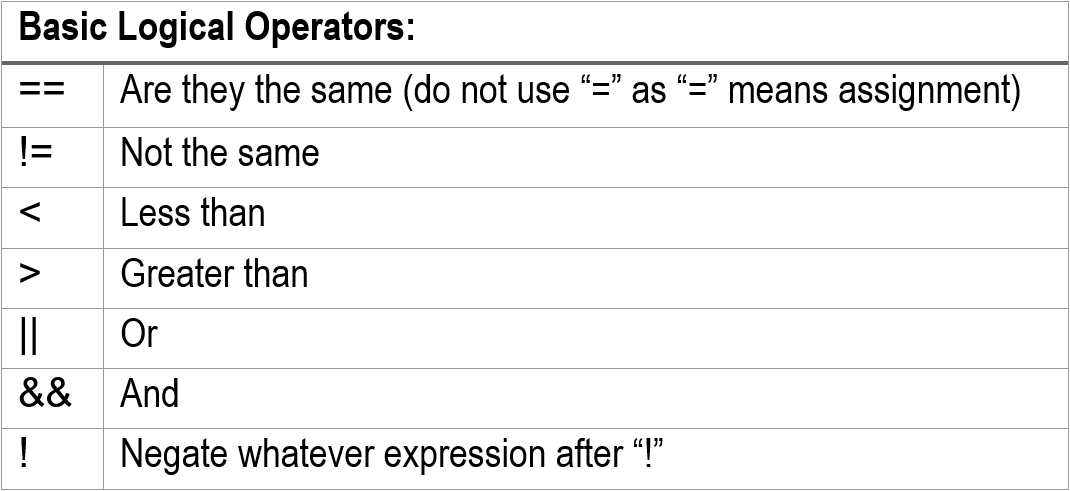# Using Booleans in VEXcode Pro V5

The “bool” data type generates logically true or false.

##### Logical/Boolean Operators##### Logical/Boolean Expressions
```if(<boolean expressions>)
{
.....<block>
}
```

The `<boolean expressions>` will contain a single or complex expression to be evaluated. The `<block>` means a block of code that will be executed only if the `<boolean expressions>` are evaluated to be true.

##### More Boolean Expressions:
 Boolean Expression What it means if (x == 10) if x is equal to 10 if (x <= 10) if x is less than and equal to 10 if (x > 10 || y > 20) if x is greater than 10 or y is greater than 20 if (x <= 10 && y <= 20) if x<=10 and y<=20 if !(x > 10 || y > 20) if x<=10  and y<=20 if ( !( x <=10 || x >=20) ) If x>10 and x<20

## Use special caution when using boolean expressions!

A Boolean type (bool) is a simple integer value.

Let’s take a look at how `if (... )` is interpreted:

• `if (...)` will be computed by the compiler; it produces a meaning of true or false.
• Truth is: when ( ... ) produces anything other than 0 (i.e. zero), the `if ( .... )` will mean true.
• So: the following expressions are always true:
• if (1)
• if ( 10 )
• if ( anything results non-zero)

Common Errors you must pay attention to:

Example 1:

```int X = 10, Y=20;
if  (X = Y)
brain.Screen.print("X and Y are the same.”);
else
brain.Screen.print("X and Y are different.”);
```

Output: X and Y are the same.

Why?

`if (X = Y)` really means:

• Assign Y to X, so X has value of 20
• The compiler interprets it as if (20) where (20) is true as it is not (0).

Example 2:

```int X = 0, Y=0;
if  (X = Y)
brain.Screen.print("X and Y are the same.”);
else
brain.Screen.print("X and Y are different.”);
```

Output: X and Y are different.

Why?

`if (X = Y)` really means:

• Assign Y to X, so X has value of 0 (zero).
• Compiler interprets it as: (0) as false.